## What is Maximum Likelihood?

Given a probability distribution, you want to estimate the parameters of the distribution. Recall that a Normal distribution has two parameters: mean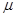and variance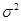. Mean is the average of the data which measures the central tendency of the data. Variance is the average of square deviation of the data from the mean.

There are many ways to estimate the parameter of a distribution. One of the most well-known method to estimate the parameter of a distribution is called Maximum Likelihood method as proposed by well -known statistician R. A. Fisher in 1912. The method is to form a likelihood function from the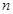sample data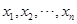and then take the partial derivative with respect to its parameters and set it to zero. The likelihood function is the product of the probability density function of all sample data.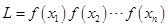Let us take an example of a Normal distribution which probability density function is given as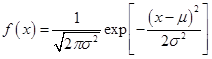Forsample data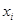, the likelihood function is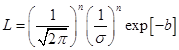where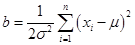Taking the logarithm to the likelihood function gives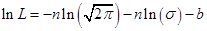Taking the partial derivative with respect to the mean, we have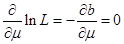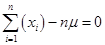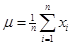Taking the partial derivative with respect to the standard deviation, we have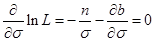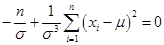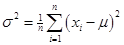Observed that given a probability density function, we can use calculus to find the formula parameters of the distribution.

For Gaussian Mixture distribution, however, the likelihood function is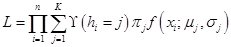Function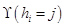produces 1 if the datais belong to component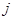and zero otherwise. The weights of componentis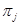. The Normal density function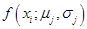of datais now depends on component. To use calculus to solve the partial derivative is very difficult. A numerical method is needed. Numerical method will not give you the formula of the parameters but it will give you the values of the parameters.

### Summary

Let us summarize what you have learned in this section:

• Maximum Likelihood method is useful to find the parameters of a distribution.
• For GMM, maximum likelihood method using partial derivative is too difficult. We need numerical solution.

In the next section, you will learn an algorithm to solve GMM numerically .

.

Preferable reference for this tutorial is

Teknomo, Kardi. (2019) Gaussian Mixture Model and EM Algorithm in Microsoft Excel.
http://people.revoledu.com/kardi/tutorial/EM/